# Sequence Alignments Revisited Scoring nucleotide sequence alignments was

• Slides: 10Sequence Alignments Revisited · Scoring nucleotide sequence alignments was easier • Match score • Possibly different scores for transitions and transversions · For amino acids, there are many more possible substitutions · How do we score which substitutions are highly penalized and which are moderately penalized? • Physical and chemical characteristics • Empirical methods Protein-Related Algorithms Intro to Bioinformatics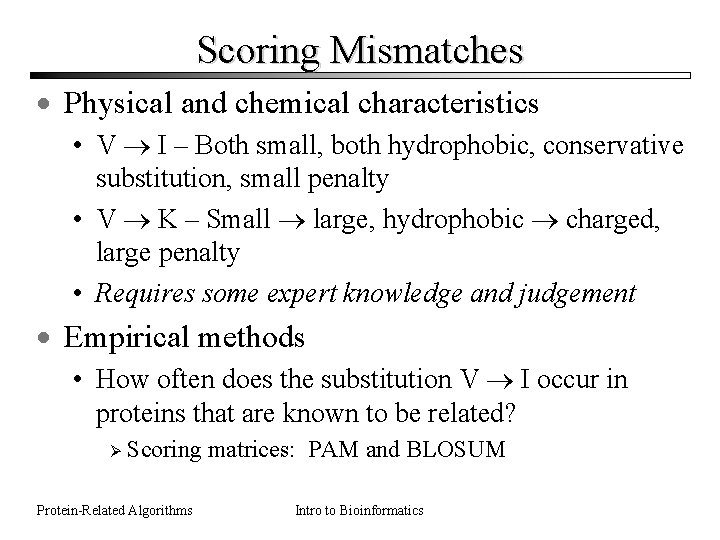Scoring Mismatches · Physical and chemical characteristics • V I – Both small, both hydrophobic, conservative substitution, small penalty • V K – Small large, hydrophobic charged, large penalty • Requires some expert knowledge and judgement · Empirical methods • How often does the substitution V I occur in proteins that are known to be related? Ø Scoring matrices: PAM and BLOSUM Protein-Related Algorithms Intro to Bioinformatics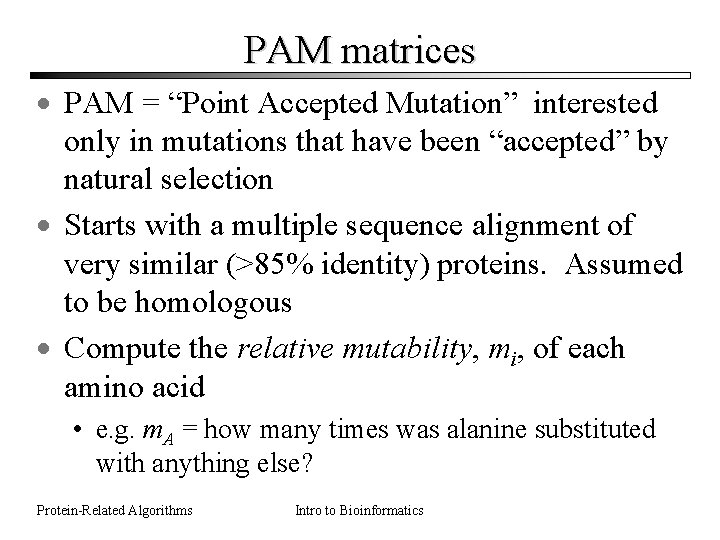PAM matrices · PAM = “Point Accepted Mutation” interested only in mutations that have been “accepted” by natural selection · Starts with a multiple sequence alignment of very similar (>85% identity) proteins. Assumed to be homologous · Compute the relative mutability, mi, of each amino acid • e. g. m. A = how many times was alanine substituted with anything else? Protein-Related Algorithms Intro to Bioinformatics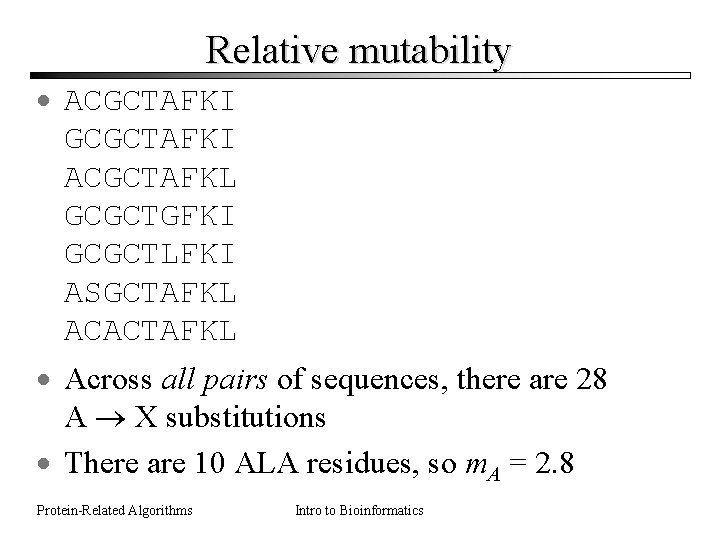Relative mutability · ACGCTAFKI GCGCTAFKI ACGCTAFKL GCGCTGFKI GCGCTLFKI ASGCTAFKL ACACTAFKL · Across all pairs of sequences, there are 28 A X substitutions · There are 10 ALA residues, so m. A = 2. 8 Protein-Related Algorithms Intro to BioinformaticsPam Matrices, cont’d · Construct a phylogenetic tree for the sequences in the alignment FG, A = 3 · Calculate substitution frequences FX, X · Substitutions may have occurred either way, so A G also counts as G A. Protein-Related Algorithms Intro to BioinformaticsMutation Probabilities · Mi, j represents the probability of J I substitution. · Protein-Related Algorithms = 2. 025 Intro to Bioinformatics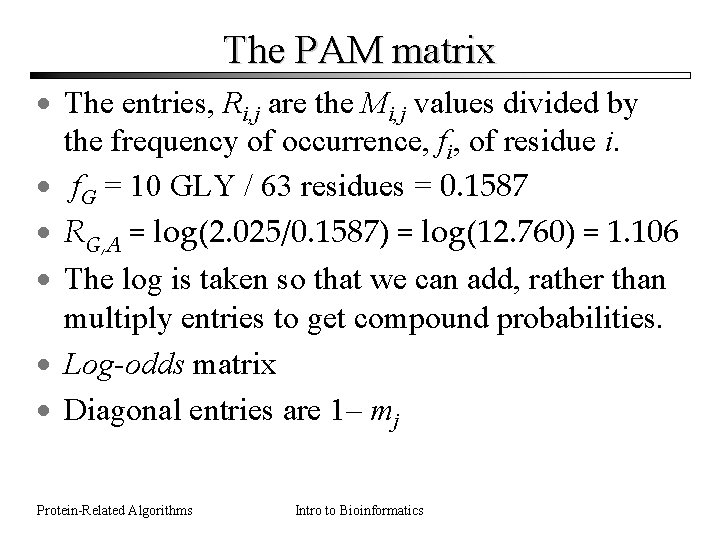The PAM matrix · The entries, Ri, j are the Mi, j values divided by the frequency of occurrence, fi, of residue i. · f. G = 10 GLY / 63 residues = 0. 1587 · RG, A = log(2. 025/0. 1587) = log(12. 760) = 1. 106 · The log is taken so that we can add, rather than multiply entries to get compound probabilities. · Log-odds matrix · Diagonal entries are 1– mj Protein-Related Algorithms Intro to Bioinformatics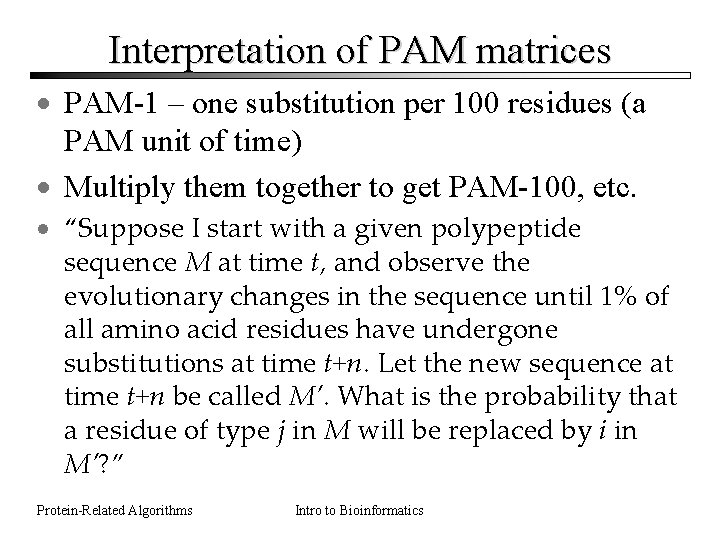Interpretation of PAM matrices · PAM-1 – one substitution per 100 residues (a PAM unit of time) · Multiply them together to get PAM-100, etc. · “Suppose I start with a given polypeptide sequence M at time t, and observe the evolutionary changes in the sequence until 1% of all amino acid residues have undergone substitutions at time t+n. Let the new sequence at time t+n be called M’. What is the probability that a residue of type j in M will be replaced by i in M’? ” Protein-Related Algorithms Intro to Bioinformatics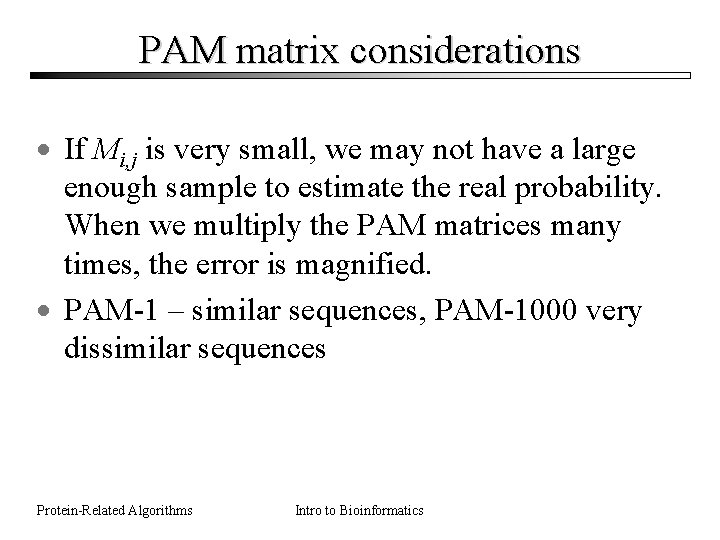PAM matrix considerations · If Mi, j is very small, we may not have a large enough sample to estimate the real probability. When we multiply the PAM matrices many times, the error is magnified. · PAM-1 – similar sequences, PAM-1000 very dissimilar sequences Protein-Related Algorithms Intro to BioinformaticsBLOSUM matrix · Starts by clustering proteins by similarity · Avoids problems with small probabilities by using averages over clusters · Numbering works opposite • BLOSUM-62 is appropriate for sequences of about 62% identity, while BLOSUM-80 is appropriate for more similar sequences. Protein-Related Algorithms Intro to Bioinformatics